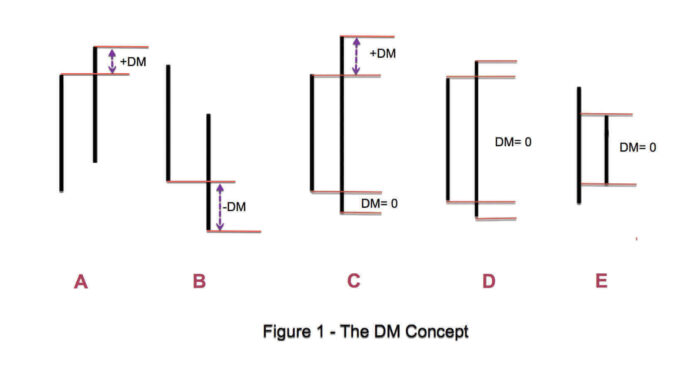# Directional Movement Index (DMI)

0
30You are here:
Estimated reading time: 1 min

The Directional Movement Index (or DMI) was developed by J. Welles Wilder in order to determine the overall direction of an asset’s price.

This technical indicator helps traders assess the trend direction by comparing prior highs and lows

DMI is composed of two lines:

1. A positive directional movement line (+DI)
2. A negative directional movement line (-DI).

An optional third line, called directional movement (DX) shows the difference between the lines.

• If +DI is above -DI, there is more upward pressure than downward pressure in the price.
• If -DI is above +DI, then there is more downward pressure on the price.

Crossovers between the lines are also sometimes used as trade signals to buy or sell.

For example, the +DI crossing above the -DI may signal the start of an uptrend in price.

The larger the spread between the two lines, the stronger the price trend.

• If +DI is way above -DI the price trend is strongly up.
• If -DI is way above +DI then the price trend is strongly down.

#### Calculation

To calculate the DMI, you calculate the difference between the current high and the previous high (“HiDiff”), as well as the difference between the previous low and the current low (“LowDiff”).

HiDiff and LowDiff are then compared.

• If HiDiff is greater in value, a variable +DMI is set to HiDiff, and a variable -DMI is set to 0.
• If LowDiff is greater, -DMI is set to LowDiff, and +DMI is set to 0.
• If the two values are equal, or if no trend is seen in either highs or lows, both values are set to 0.

A calculation known as the Welles Summation is then performed on both +DMI and -DMI, resulting in two numbers: +DI and -DI, both ranging from 0 to 100.

If you liked this article, then please subscribe to our Newsletter Services for Forex Related updates. You can also find us on Facebook. and can join our Community Portal. If you have any confusion please leave your comments below.